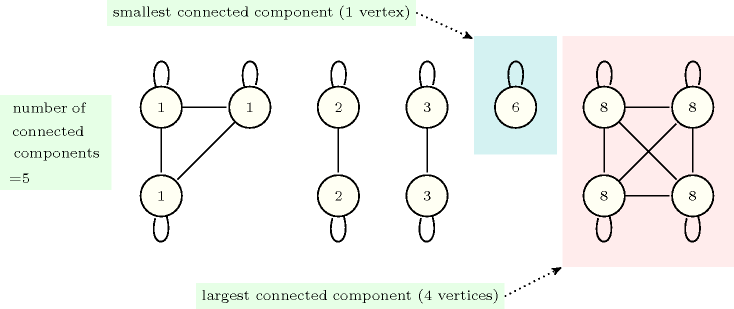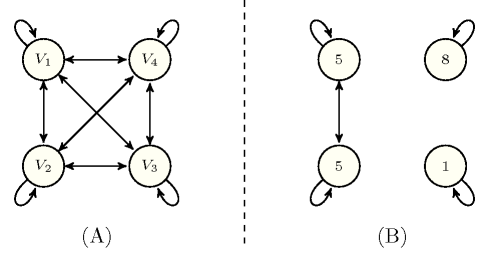### 2.3.1. Basic ideas and illustrative example

Within the graph-based representation, a global constraint is represented as a digraph where each vertex corresponds to a variable and each arc to a binary arc constraint between the variables associated with the extremities of the corresponding arc. The main difference with classical constraint networks [DechterPearl87], stems from the fact that we do not force any more all arc constraints to hold. We rather consider this graph from which we discard all the arc constraints that do not hold as well as all isolated vertices (i.e, vertices not involved any more in any arc) and impose one or several graph properties on this remaining graph. These properties can for instance be a restriction on the number of connected components, on the size of the smallest connected component or on the size of the largest connected component.

##### Figure 2.3.1. Illustration of the link between graph-properties and global constraintsEXAMPLE: We give an example of interpretation of such graph properties in terms of global constraints. For this purpose we consider the sequence $s$ of values $1311288236883$ from which we construct the following graph $G$:

• To each value associated with a position in $s$ corresponds a vertex of $G$,

• There is an arc from a vertex ${v}_{1}$ to a vertex ${v}_{2}$ if these vertices correspond to the same value.

Figure 2.3.1 depicts graph $G$. Since $G$ is symmetric, we omit the directions of the arcs. We have the following correspondence between graph properties and constraints on the sequence $s$:

• The number of connected components of $G$ corresponds to the number of distinct values of $s$.

• The size of the smallest connected component of $G$ is the smallest number of occurrences of the same value in $s$.

• The size of the largest connected component of $G$ is the largest number of occurrences of the same value in $s$.

As a result, in this context, putting a restriction on the number of connected components of $G$ can been seen as a global constraint on the number of distinct values of a sequence of variables. Similar global constraints can be associated with the two other graph properties.

We now explain how to generate the initial graph associated with a global constraint. A global constraint has one or more arguments, which usually correspond to an integer value, to one variable or to a collection of variables. Therefore we have to describe the process that allows for generating the vertices and the arcs of the initial graph from the arguments of a global constraint under consideration. For this purpose we will take a concrete example.

Consider the constraint $\mathrm{𝚗𝚟𝚊𝚕𝚞𝚎}$$\left(\mathrm{𝙽𝚅𝙰𝙻},\mathrm{𝚅𝙰𝚁𝙸𝙰𝙱𝙻𝙴𝚂}\right)$ where $\mathrm{𝙽𝚅𝙰𝙻}$ and $\mathrm{𝚅𝙰𝚁𝙸𝙰𝙱𝙻𝙴𝚂}$ respectively correspond to a domain variable and to a collection of domain variables $〈\mathrm{𝚟𝚊𝚛}-{V}_{1},\mathrm{𝚟𝚊𝚛}-{V}_{2},\cdots ,\mathrm{𝚟𝚊𝚛}-{V}_{m}〉$.$\mathrm{𝚟𝚊𝚛}$ corresponds to the name of the attribute used in the collection of variables. This constraint holds if $\mathrm{𝙽𝚅𝙰𝙻}$ is equal to the number of distinct values assigned to the variables ${V}_{1},{V}_{2},\cdots ,{V}_{m}$. We first show how to generate the initial graph associated with the $\mathrm{𝚗𝚟𝚊𝚕𝚞𝚎}$ constraint. We then describe the arc constraint associated with each arc of this graph. Finally, we give the graph property we impose on the final graph.

To each variable of the collection $\mathrm{𝚅𝙰𝚁𝙸𝙰𝙱𝙻𝙴𝚂}$ corresponds a vertex of the initial graph. We generate an arc between each pair of vertices. To each arc, we associate an equality constraint between the variables corresponding to the extremities of that arc. We impose that $\mathrm{𝙽𝚅𝙰𝙻}$, the variable corresponding to the first argument of $\mathrm{𝚗𝚟𝚊𝚕𝚞𝚎}$, be equal to the number of strongly connected components of the final graph. This final graph consists of the initial graph from which we discard all arcs such that the corresponding equality constraint does not hold.

Part (A) of Figure 2.3.2 shows the graph initially generated for the constraint $\mathrm{𝚗𝚟𝚊𝚕𝚞𝚎}$

$\left(\mathrm{𝙽𝚅𝙰𝙻},〈\mathrm{𝚟𝚊𝚛}-{V}_{1},\mathrm{𝚟𝚊𝚛}-{V}_{2},\mathrm{𝚟𝚊𝚛}-{V}_{3},\mathrm{𝚟𝚊𝚛}-{V}_{4}〉\right)$, where $\mathrm{𝙽𝚅𝙰𝙻}$, ${V}_{1}$, ${V}_{2}$, ${V}_{3}$ and ${V}_{4}$ are domain variables. Part (B) presents the final graph associated with the ground instance $\mathrm{𝚗𝚟𝚊𝚕𝚞𝚎}$$\left(3,〈\mathrm{𝚟𝚊𝚛}-5,\mathrm{𝚟𝚊𝚛}-5,\mathrm{𝚟𝚊𝚛}-1,\mathrm{𝚟𝚊𝚛}-8〉\right)$. For each vertex of the initial and final graph we respectively indicate the corresponding variable and the value assigned to that variable. We have removed from the final graph all the arcs associated with equalities that do not hold. The constraint $\mathrm{𝚗𝚟𝚊𝚕𝚞𝚎}$$\left(3,〈\mathrm{𝚟𝚊𝚛}-5,\mathrm{𝚟𝚊𝚛}-5,\mathrm{𝚟𝚊𝚛}-1,\mathrm{𝚟𝚊𝚛}-8〉\right)$ holds since the final graph contains three strongly connected components, which in the context of the definition of the $\mathrm{𝚗𝚟𝚊𝚕𝚞𝚎}$ constraint, can be reinterpreted as the fact that $\mathrm{𝙽𝚅𝙰𝙻}$ is the number of distinct values assigned to variables ${V}_{1},{V}_{2},{V}_{3},{V}_{4}$.

##### Figure 2.3.2. (A) Initial and (B) final graph associated with the constraint $\mathrm{𝚗𝚟𝚊𝚕𝚞𝚎}$$\left(3,〈\mathrm{𝚟𝚊𝚛}-5,\mathrm{𝚟𝚊𝚛}-5,\mathrm{𝚟𝚊𝚛}-1,\mathrm{𝚟𝚊𝚛}-8〉\right)$Now that we have illustrated the basic ideas for describing a global constraint in terms of graph properties, we go into more details.